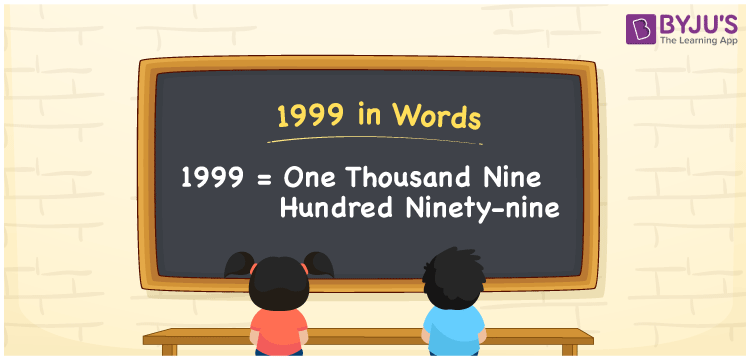# 1999 in words

1999 in words is written as One Thousand Nine Hundred and Ninety Nine. 1999 represents the count or value. The article on Counting Numbersc an give you an idea about count or counting. The number 1999 is used in expressions that relate to money, distance, length, year and others. Let us consider an example for 1999. “Reena was born in the year 1999”. Another example, “On a trek I recorded One Thousand Nine Hundred and Ninety Nine steps on my smart watch.”

 1999 in words One Thousand Nine Hundred and Ninety Nine One Thousand Nine Hundred and Ninety Nine in Numbers 1999

## 1999 in English Words## How to Write 1999 in Words?

We can convert 1999 to words using a place value chart. The number 1999 has 4 digits, so let’s make a chart that shows the place value up to 4 digits.

 Thousands Hundreds Tens Ones 1 9 9 9

Thus, we can write the expanded form as:

1 × Thousand + 9 × Hundred + 9 × Ten + 9 × One

= 1 × 1000 + 9 × 100 + 9 × 10 + 9 × 1

= 1999

= One Thousand Nine Hundred and Ninety Nine.

1999 is the natural number that is succeeded by 1998 and preceded by 2000.

1999 in words – One Thousand Nine Hundred and Ninety Nine.

Is 1999 an odd number? – Yes.

Is 1999 an even number? – No.

Is 1999 a perfect square number? – No.

Is 1999 a perfect cube number? – No.

Is 1999 a prime number? – Yes.

Is 1999 a composite number? – No.

## Solved Example

1. Write the number 1999 in expanded form

Solution: 1 × 1000 + 9 × 100 + 9 × 10 + 9 × 1

We can write 1999 = 1000 + 900 + 90 + 9

= 1 × 1000 + 9 × 100 + 9 × 10 + 9 × 1

## Frequently Asked Questions on 1999 in words

Q1

### How to write 1999 in words?

1999 in words is written as One Thousand Nine Hundred and Ninety Nine.
Q2

### State True or False. 1999 is divisible by 2?

False. 1999 is not divisible by 2.
Q3

### Is 1999 divisible by 10?

No. 1999 is not divisible by 10.